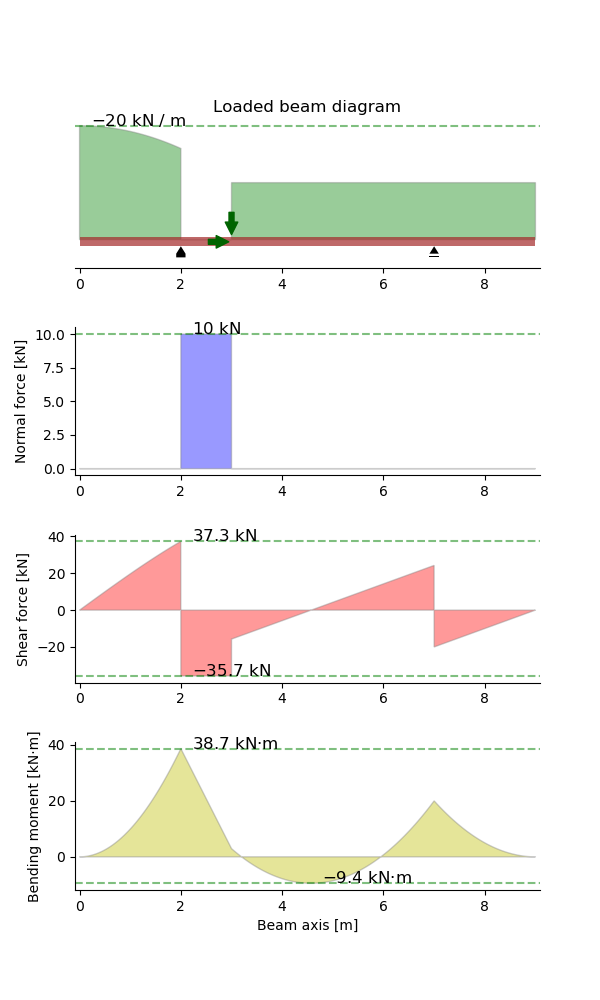This example demonstrates some functionality of the beambending package.

## Specifications¶

A beam resting on two supports at x=2 m and x=7 m, with the following applied loads:

• a downward force of 20 kN at x=3 m;
• a force of 10 kN pointing right, also at x=3 m;
• a downward distributed load of 20 kN/m on 0 <= x <= 2 m;
• a downward distributed load of 10 kN/m on 3 m <= x <= 9 m

## Results¶

The figure below shows the load case corresponding to the description above. The resulting diagrams are respectively:

1. a schematic of the beam and its applied (external) loads  ,
2. a normal force diagram: $$\mathbf{N(x)}$$,
3. a shear force diagram $$\mathbf{V(x)}$$, and
4. a bending moment diagram $$\mathbf{M(x)}$$.
  The x-coordinates of the point loads are marked with green arrows, but magnitudes are not displayed in the beam schematic in order to keep the figure clean and simple.## Code¶

# -*- coding: utf-8 -*-
"""Example 1: Shear force and bending moment diagrams for a beam subjected to
"""
import os

def example_1():
"""Run example 1"""
beam = Beam(9)  # Initialize a Beam object of length 9 m
beam.pinned_support = 2    # x-coordinate of the pinned support
beam.rolling_support = 7  # x-coordinate of the rolling support
PointLoadH(10, 3),  # 10 kN pointing right, at x=3 m
PointLoadV(-20, 3),  # 20 kN downwards, at x=3 m
DistributedLoadV(-10, (3, 9)),  # 10 kN/m, downwards, for 3 m <= x <= 9 m
DistributedLoadV(-20 + x**2, (0, 2)),  # variable load, for 0 <= x <= 2 m
))
fig = beam.plot()

# save the png and add it to the documentation
mod_path = os.path.dirname(os.path.abspath(__file__))  # current module
save_name = os.path.basename(__file__).replace('.py', '.png')  # file name
save_path = os.path.join(mod_path, save_name)
fig.savefig(save_path, transparent=True)

if __name__ == '__main__':  # call function when run as script
example_1()